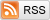# Visualizing DBSCAN Clustering

January 24, 2015

A previous post covered clustering with the k-means algorithm. In this post, we consider a fundamentally different, density-based approach called DBSCAN. In contrast to k-means, which modeled clusters as sets of points near to their center, density-based approaches like DBSCAN model clusters as high-density clumps of points. To begin, choose a data set below:

DBSCAN, (Density-Based Spatial Clustering of Applications with Noise), captures the insight that clusters are dense groups of points. The idea is that if a particular point belongs to a cluster, it should be near to lots of other points in that cluster.

It works like this: First we choose two parameters, a positive number epsilon and a natural number minPoints. We then begin by picking an arbitrary point in our dataset. If there are more than minPoints points within a distance of epsilon from that point, (including the original point itself), we consider all of them to be part of a "cluster". We then expand that cluster by checking all of the new points and seeing if they too have more than minPoints points within a distance of epsilon, growing the cluster recursively if so.

Eventually, we run out of points to add to the cluster. We then pick a new arbitrary point and repeat the process. Now, it's entirely possible that a point we pick has fewer than minPoints points in its epsilon ball, and is also not a part of any other cluster. If that is the case, it's considered a "noise point" not belonging to any cluster.

(There's a slight complication worth pointing out: say minPoints=4, and you have a point with three points in its epsilon ball, including itself. Say the other two points belong to two different clusters, and each has 4 points in their epsilon balls. Then both of these dense points will "fight over" the original point, and it's arbitrary which of the two clusters it ends up in. To see what I mean, try out "Example A" with minPoints=4, epsilon=1.98. Since DBSCAN considers the points in an arbitrary order, the middle point can end up in either the left or the right cluster on different runs. This kind of point is known as a "border point").

To illustrate the "epsilon ball rules", before the algorithm runs I superimpose a grid of epsilon balls over the dataset you choose, and color them in if they contain more than minPoints points. To get an additional feel for how this algorithm works, check out the "DBSCAN Rings" dataset, which consists of different numbers of points in different sized circles.

Note that in the actual DBSCAN algorithm, epsilon and minPoints remain the same throughout. But I thought it'd be fun to play around with changing them while the algorithm is running, so I've left the option in to do so.

Take a look at how the k-means algorithm performs on these same datasets. How does it compare? Where is it better and where is it worse?